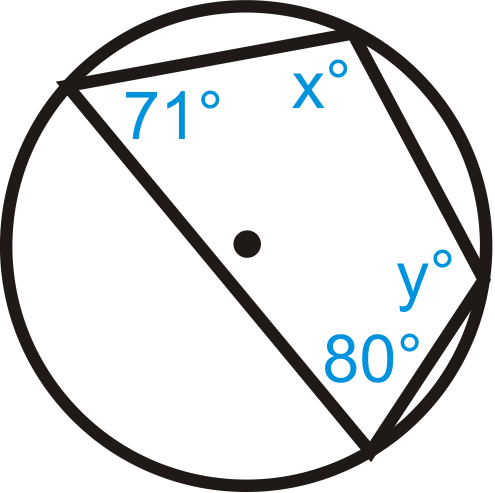# 6.15: Inscribed Quadrilaterals in Circles

•• Contributed by CK12
• CK12

Quadrilaterals with every vertex on a circle and opposite angles that are supplementary.

An inscribed polygon is a polygon where every vertex is on the circle, as shown below.Figure $$\PageIndex{1}$$

For inscribed quadrilaterals in particular, the opposite angles will always be supplementary.

Inscribed Quadrilateral Theorem: A quadrilateral can be inscribed in a circle if and only if the opposite angles are supplementary.Figure $$\PageIndex{2}$$

If $$ABCD$$ is inscribed in $$\bigodot E$$, then $$m\angle A+m\angle C=180^{\circ}$$ and $$m\angle B+m\angle D=180^{\circ}$$. Conversely, If $$m\angle A+m\angle C=180^{\circ}$$ and $$m\angle B+m\angle D=180^{\circ}$$, then $$ABCD$$ is inscribed in $$\bigodot E$$.

What if you were given a circle with a quadrilateral inscribed in it? How could you use information about the arcs formed by the quadrilateral and/or the quadrilateral's angle measures to find the measure of the unknown quadrilateral angles?

Example $$\PageIndex{1}$$

1.Figure $$\PageIndex{3}$$
2.Figure $$\PageIndex{4}$$

Solution

1. \begin{aligned} x+80^{\circ}&=180^{\circ} \qquad& y+71^{\circ}&=180^{\circ} \\ x&=100^{\circ} & y&=109^{\circ} \end{aligned}
1. \begin{aligned} z+93^{\circ} &=180^{\circ} & x&=\frac{1}{2}\left(58^{\circ}+106^{\circ}\right) & y+82^{\circ}&=180^{\circ} \\ z &=87^{\circ} & x &=82^{\circ} & y&=98^{\circ} \end{aligned}

Example $$\PageIndex{2}$$

Find $$x$$ and $$y$$ in the picture below.Figure $$\PageIndex{5}$$

Solution

$$\begin{array}{rlrl} (7 x+1)^{\circ}+105^{\circ} & =180^{\circ} & (4 y+14)^{\circ}+(7 y+1)^{\circ} & =180^{\circ} \\ 7 x+106^{\circ} & =180^{\circ} & 11 y+15^{\circ} & =180^{\circ} \\ 7 x & =74 & 11 y & =165 \\ x & =10.57 & y&=15 \end{array}$$

Example $$\PageIndex{3}$$

Find the values of x and y in $$\bigodot A$$.Figure $$\PageIndex{6}$$

Solution

Use the Inscribed Quadrilateral Theorem. $$x^{\circ}+108^{\circ}=180^{\circ}$$ so $$x=72^{\circ}$$. Similarly, $$y^{\circ}+88^{\circ}=180^{\circ}$$ so $$y=92^{\circ}$$.

Example $$\PageIndex{4}$$

Quadrilateral $$ABCD$$ is inscribed in $$\bigodot E$$. Find $$m\angle A$$, $$m\angle B$$, $$m\angle C$$, and $$m\angle D$$.Figure $$\PageIndex{7}$$

Solution

First, note that $$m\widehat{AD}=105^{\circ}$$ because the complete circle must add up to $$360^{\circ}$$.

\begin{aligned}m\angle A&=\dfrac{1}{2}m\widehat{BD}=12(115+86)=100.5^{\circ} \\ m\angle B&=\dfrac{1}{2}m\widehat{AC}=12(86+105)=95.5^{\circ} \\ m\angle C&=180^{\circ}−m\angle A=180^{\circ}−100.5^{\circ}=79.5^{\circ} \\ m\angle D&=180^{\circ}−m\angle B=180^{\circ}−95.5^{\circ}=84.5^{\circ}\end{aligned}

## Review

Fill in the blanks.

1. A(n) _______________ polygon has all its vertices on a circle.
2. The _____________ angles of an inscribed quadrilateral are ________________.

Quadrilateral $$ABCD$$ is inscribed in $$\bigodot E$$. Find:Figure $$\PageIndex{8}$$
1. $$m\angle DBC$$
2. $$m\widehat{BC}$$
3. $$m\widehat{AB}$$
4. $$m\angle ACD$$
5. $$m\angle ADC$$
6. $$m\angle ACB$$

Find the value of $$x$$ and/or $$y$$ in $$\bigodot A$$.

1.Figure $$\PageIndex{9}$$
2.Figure $$\PageIndex{10}$$
3.Figure $$\PageIndex{11}$$

Solve for $$x$$.

1.Figure $$\PageIndex{12}$$
2.Figure $$\PageIndex{13}$$

## Vocabulary

Term Definition
central angle An angle formed by two radii and whose vertex is at the center of the circle.
chord A line segment whose endpoints are on a circle.
circle The set of all points that are the same distance away from a specific point, called the center.
diameter A chord that passes through the center of the circle. The length of a diameter is two times the length of a radius.
inscribed angle An angle with its vertex on the circle and whose sides are chords.
intercepted arc The arc that is inside an inscribed angle and whose endpoints are on the angle.
radius The distance from the center to the outer rim of a circle.
Inscribed Polygon An inscribed polygon is a polygon with every vertex on a given circle.
Inscribed Quadrilateral Theorem The Inscribed Quadrilateral Theorem states that a quadrilateral can be inscribed in a circle if and only if the opposite angles of the quadrilateral are supplementary.

Interactive Element

Video: Inscribed Quadrilaterals in Circles Principles - Basic

Activities: Inscribed Quadrilaterals in Circles Discussion Questions

Study Aids: Inscribed in Circles Study Guide